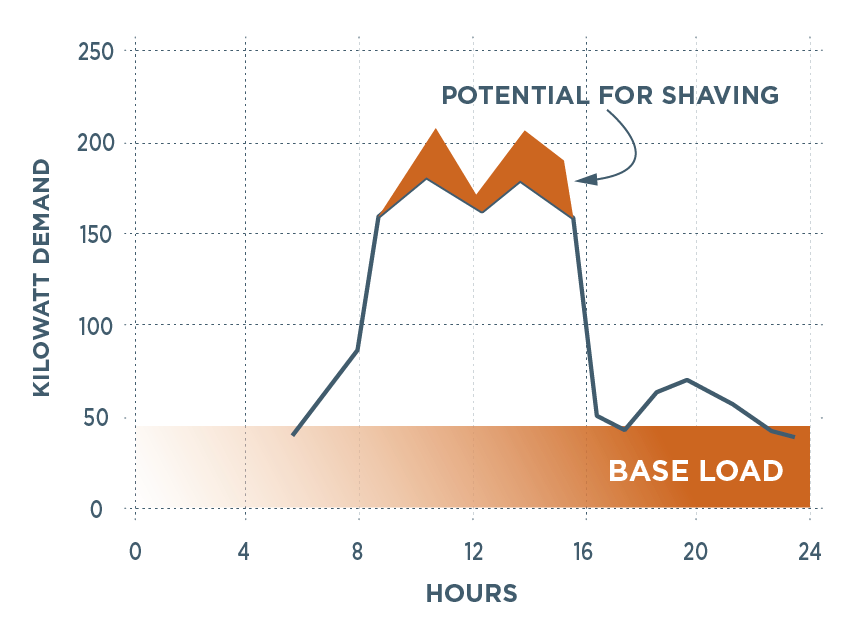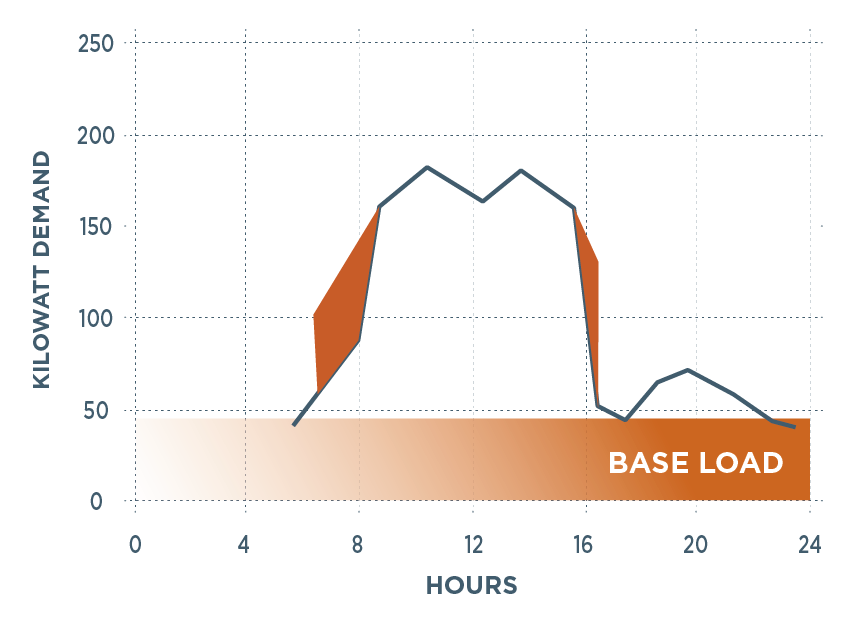Load factor is the actual amount of kilowatt hours (kWh) delivered on a system in a designated period, as opposed to the total possible kWh that could be delivered on a system. Increasing your load factor will reduce the average unit cost of the kWh. In many situations, improving load factor could save you money.

Your company or commercial institution could lower its demand by improving load factor.

Increasing your load factor will diminish the average unit cost (demand and energy) of the kWh. Depending on your situation, improving your load factor could mean substantial savings.

The load factor corresponds to the ratios between your actual energy consumption (kWh) and the maximum power recorded (demand) for that period of time.

Consumption during the period (kWh) x 100
Demand x Number of hours in that period.

A – Demand reduction

B – Increase production

Keeping the demand stable and increasing your consumption is often a cost-effective way to increase production while maximizing the use of your power.

*In both cases, the load factor will improve and therefore reduce your average unit cost per kWh.

## How to calculate LOAD FACTOR?

The load factor percentage is derived by dividing the total kilowatt-hours (kWh) consumed in a designated period by the product of the maximum demand in kilowatts (kW) and the number of hours in the period. In the example below, the monthly kWh consumption is 36,000 and the peak demand is 100 kW. There were 30 days in the billing period.

To determine the load factor, you can use the following formula:

Total kWh for the billing period x 100
(Peak Demand X # of Days X 24 Hours)

EXAMPLE:

This load factor indicates the monthly energy consumption of 36,000 kWh used by the customer was 50% of the total energy available (72,000 kWh) for use at the 80 kW level.

To determine the load factor, you can use the following formula:

Total kWh for the billing period x 100
(Peak Demand X # of Days X 24 Hours)

________36, 000 kWh_x 100___
80 kW x 30 days x 24 hrs/day

36,000 kWh x 100
57,600 kWh

= 0.625 x 100

ANOTHER EXAMPLE:

Total kWh = 18,000 kWh

Demand = 50 kW

The number of Days = 30

Hours per Day = 24

______18, 000 kWh__x 100_____
(50 kW X 30 Days X 24 Hours)

18,000 kWh x 100
36,000 kWh

= 0.50 x 100

= 50%

In this example, the Load Factor is 50% showing that, on average, the peak demand was fully used for 12 hours a day for 30 days. One of the simplest ways of improving load factor is to “shave the peaks”. “Shaving” means having a portion of the electrical load now operating at peak times of the day shifted to non-peak times.

For example, instead of operating ten machines at 11:00 a.m., it would be advantageous to have three operating at 9:00 a.m., five at 10:00 a.m. and two at 11:30 p.m. All machines are still operating but not at the same time.

The diagram below indicated in red, where savings would be possible;The diagram below shows, the daily load profile after the peaks have been shaved. The dark shaded peak area above has been shaved and relocated in the illustration below.